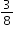# MA.8.DP.2.3

Solve real-world problems involving probabilities related to single or repeated experiments, including making predictions based on theoretical probability.

### Examples

Example: If Gabriella rolls a fair die 300 times, she can predict that she will roll a 3 approximately 50 times since the theoretical probability is.

Example: Sandra performs an experiment where she flips a coin three times. She finds the theoretical probability of landing on exactly one head as. If she performs this experiment 50 times (for a total of 150 flips), predict the number of repetitions of the experiment that will result in exactly one of the three flips landing on heads.

### Clarifications

Clarification 1: Instruction includes making connections to proportional relationships and representing probability as a fraction, percentage or decimal.

Clarification 2: Experiments to be repeated are limited to tossing a fair coin, rolling a fair die, picking a card randomly from a deck with replacement, picking marbles randomly from a bag with replacement and spinning a fair spinner.

Clarification 3: Repetition of experiments is limited to two times except for tossing a coin.

General Information
Subject Area: Mathematics (B.E.S.T.)
Strand: Data Analysis and Probability
Date Adopted or Revised: 08/20
Status: State Board Approved

## Related Courses

This benchmark is part of these courses.
1200400: Foundational Skills in Mathematics 9-12 (Specifically in versions: 2014 - 2015, 2015 - 2022, 2022 and beyond (current))
1205050: M/J Accelerated Mathematics Grade 7 (Specifically in versions: 2014 - 2015, 2015 - 2020, 2020 - 2022, 2022 and beyond (current))
1205070: M/J Grade 8 Pre-Algebra (Specifically in versions: 2014 - 2015, 2015 - 2022, 2022 and beyond (current))
1204000: M/J Foundational Skills in Mathematics 6-8 (Specifically in versions: 2014 - 2015, 2015 - 2022, 2022 and beyond (current))
7812030: Access M/J Grade 8 Pre-Algebra (Specifically in versions: 2014 - 2015, 2015 - 2018, 2018 - 2019, 2019 - 2022, 2022 and beyond (current))

## Related Access Points

Alternate version of this benchmark for students with significant cognitive disabilities.
MA.8.DP.2.AP.3: Compare actual results of an experiment with its theoretical probability (e.g., make a statement that describes the relationship between the actual results of an experiment with its theoretical probability [e.g., more, less, same, different, equal]).

## Related Resources

Vetted resources educators can use to teach the concepts and skills in this benchmark.

## Formative Assessments

Coat Count:

Students are asked to design a simulation to generate frequencies for complex events.

Type: Formative Assessment

Probabilities Cubed:

Students are asked to estimate the frequency of an event given its probability and explain why an expected frequency might differ from an observed frequency.

Type: Formative Assessment

## Lesson Plans

Independent Compound Probability:

During this lesson, students will use Punnett Squares to determine the probability of an offspring's characteristics.

Type: Lesson Plan

Understanding Probability of Compound Events:

This lesson uses guided teaching, small group activities, and student creations all-in-one! Students will be able to solve and create compound event word problems. They will also be able to identify what type of event is being used in a variety of word problems.

Type: Lesson Plan

## Original Student Tutorial

Predicting Outcomes at the Carnival:

Learn how to use probability to predict expected outcomes at the Carnival in this interactive tutorial.

Type: Original Student Tutorial

## Perspectives Video: Experts

Let's Make a Math Deal:

Should I keep my choice or switch?  Learn more about the origins and probability behind the Monty Hall door picking dilemma and how Game Theory and strategy effect the probability.

Download the CPALMS Perspectives video student note taking guide.

Type: Perspectives Video: Expert

History of Probability and the Problem of Points:

What was the first question that started probability theory?

Download the CPALMS Perspectives video student note taking guide.

Type: Perspectives Video: Expert

## MFAS Formative Assessments

Coat Count:

Students are asked to design a simulation to generate frequencies for complex events.

Probabilities Cubed:

Students are asked to estimate the frequency of an event given its probability and explain why an expected frequency might differ from an observed frequency.

## Original Student Tutorials Mathematics - Grades 6-8

Predicting Outcomes at the Carnival:

Learn how to use probability to predict expected outcomes at the Carnival in this interactive tutorial.

## Student Resources

Vetted resources students can use to learn the concepts and skills in this benchmark.

## Original Student Tutorial

Predicting Outcomes at the Carnival:

Learn how to use probability to predict expected outcomes at the Carnival in this interactive tutorial.

Type: Original Student Tutorial

## Parent Resources

Vetted resources caregivers can use to help students learn the concepts and skills in this benchmark.